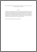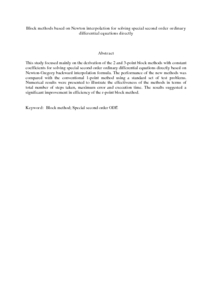# Block methods based on Newton interpolation for solving special second order ordinary differential equations directly

## Citation

Yap, Yee Ken and Ismail, Fudziah and Suleiman, Mohamed and Md. Amin, Suriah (2008) Block methods based on Newton interpolation for solving special second order ordinary differential equations directly. Journal of Mathematics and Statistics, 4 (3). pp. 174-180. ISSN 1549-3644

## Abstract

This study focused mainly on the derivation of the 2 and 3-point block methods with constant coefficients for solving special second order ordinary differential equations directly based on Newton-Gregory backward interpolation formula. The performance of the new methods was compared with the conventional 1-point method using a standard set of test problems. Numerical results were presented to illustrate the effectiveness of the methods in terms of total number of steps taken, maximum error and execution time. The results suggested a significant improvement in efficiency of the r-point block method.Preview
PDF (Abstract)
Block methods based on Newton interpolation for solving special second order ordinary differential equations directly.pdfView Item